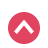We've updated our

Good job!
Take a challenge

### Generating PDF...\bold{\mathrm{Basic}} \bold{\alpha\beta\gamma} \bold{\mathrm{AB\Gamma}} \bold{\sin\cos} \bold{\ge\div\rightarrow} \bold{\overline{x}\space\mathbb{C}\forall} \bold{\sum\space\int\space\product} \bold{\begin{pmatrix}\square&\square\\\square&\square\end{pmatrix}} \bold{H_{2}O}Save to Notebook!

Verify## Number LineDescription
Rationalize complex numbers by multiplying with conjugate step-by-step

complex-numbers-conjugate-calculator

en

### Generating PDF...